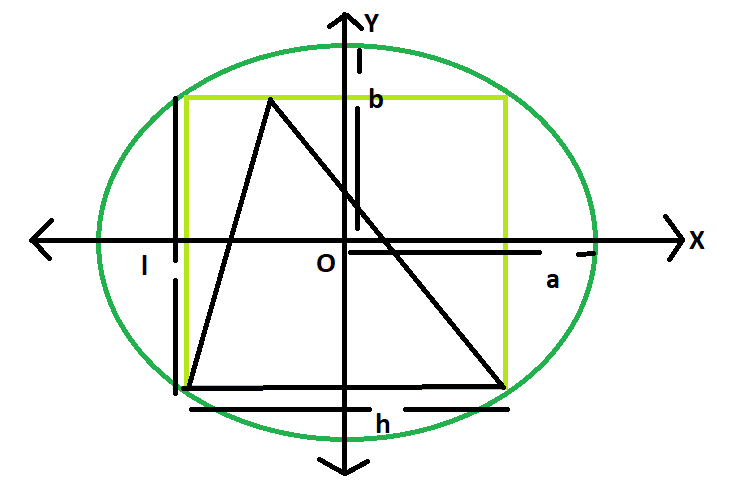GeeksforGeeks App
Open AppBrowser
Continue

# Area of a triangle inscribed in a rectangle which is inscribed in an ellipse

Given here is an ellipse with axes length 2a and 2b, which inscribes a rectangle of length l and breadth h, which in turn inscribes a triangle.The task is to find the area of this triangle.
Examples:

```Input: a = 4, b = 3
Output: 12

Input: a = 5, b = 2
Output: 10```Approach
We know the Area of the rectangle inscribed within the ellipse is, Ar = 2ab(Please refer here),
also the area of the triangle inscribed within the rectangle s, A = Ar/2 = ab(Please refer here)
Below is the implementation of the above approach:

## C++

 `// C++ Program to find the area of the triangle``// inscribed within the rectangle which in turn``// is inscribed in an ellipse``#include ``using` `namespace` `std;` `// Function to find the area of the triangle``float` `area(``float` `a, ``float` `b)``{` `    ``// length of a and b cannot be negative``    ``if` `(a < 0 || b < 0)``        ``return` `-1;` `    ``// area of the triangle``    ``float` `A = a * b;``    ``return` `A;``}` `// Driver code``int` `main()``{``    ``float` `a = 5, b = 2;``    ``cout << area(a, b) << endl;``    ``return` `0;``}`

## Java

 `   ``// Java Program to find the area of the triangle``// inscribed within the rectangle which in turn``// is inscribed in an ellipse` `import` `java.io.*;` `class` `GFG {` `// Function to find the area of the triangle``static` `float` `area(``float` `a, ``float` `b)``{` `    ``// length of a and b cannot be negative``    ``if` `(a < ``0` `|| b < ``0``)``        ``return` `-``1``;` `    ``// area of the triangle``    ``float` `A = a * b;``    ``return` `A;``}` `// Driver code`  `    ``public` `static` `void` `main (String[] args) {``    ``float` `a = ``5``, b = ``2``;``    ``System.out.println(area(a, b));``    ``}``}``//This code is contributed by anuj_67..`

## Python3

 `# Python 3 Program to find the``# area of the triangle inscribed``# within the rectangle which in``# turn is inscribed in an ellipse` `# Function to find the area``# of the triangle``def` `area(a, b):``    ` `    ``# length of a and b cannot``    ``# be negative``    ``if` `(a < ``0` `or` `b < ``0``):``        ``return` `-``1` `    ``# area of the triangle``    ``A ``=` `a ``*` `b``    ``return` `A` `# Driver code``if` `__name__ ``=``=` `'__main__'``:``    ``a ``=` `5``    ``b ``=` `2``    ``print``(area(a, b))``    ` `# This code is contributed``# by Surendra_Gangwar`

## C#

 `// C# Program to find the area of``// the triangle inscribed within``// the rectangle which in turn``// is inscribed in an ellipse``using` `System;` `class` `GFG``{``    ` `// Function to find the``// area of the triangle``static` `float` `area(``float` `a, ``float` `b)``{` `    ``// length of a and b``    ``// cannot be negative``    ``if` `(a < 0 || b < 0)``        ``return` `-1;` `    ``// area of the triangle``    ``float` `A = a * b;``    ``return` `A;``}` `// Driver code``static` `public` `void` `Main ()``{``    ``float` `a = 5, b = 2;``    ``Console.WriteLine(area(a, b));``}``}` `// This code is contributed by ajit`

## PHP

 ``

## Javascript

 ``

Output:

`10`

Time complexity: O(1)

Auxiliary Space: O(1)

My Personal Notes arrow_drop_up